Test: Problems on Pythagoras Theorem

# Test: Problems on Pythagoras Theorem - Class 10

Test Description

## 10 Questions MCQ Test Online MCQ Tests for Class 10 - Test: Problems on Pythagoras Theorem

Test: Problems on Pythagoras Theorem for Class 10 2023 is part of Online MCQ Tests for Class 10 preparation. The Test: Problems on Pythagoras Theorem questions and answers have been prepared according to the Class 10 exam syllabus.The Test: Problems on Pythagoras Theorem MCQs are made for Class 10 2023 Exam. Find important definitions, questions, notes, meanings, examples, exercises, MCQs and online tests for Test: Problems on Pythagoras Theorem below.
Solutions of Test: Problems on Pythagoras Theorem questions in English are available as part of our Online MCQ Tests for Class 10 for Class 10 & Test: Problems on Pythagoras Theorem solutions in Hindi for Online MCQ Tests for Class 10 course. Download more important topics, notes, lectures and mock test series for Class 10 Exam by signing up for free. Attempt Test: Problems on Pythagoras Theorem | 7 questions in 10 minutes | Mock test for Class 10 preparation | Free important questions MCQ to study Online MCQ Tests for Class 10 for Class 10 Exam | Download free PDF with solutions
 1 Crore+ students have signed up on EduRev. Have you?
Test: Problems on Pythagoras Theorem - Question 1

### The monitors of computers are measured along the diagonal. What is the size of the largest monitor that can be placed in a space measuring 17″ x 21″?​

Detailed Solution for Test: Problems on Pythagoras Theorem - Question 1

Since monitors are measured along the diagonals this means we need to use pythagoras theorem for this right angled triangle.
H= P+ B2
=17 * 17 + 21 * 21
=289 + 441 = 730
H=27’’ (Approx)

Test: Problems on Pythagoras Theorem - Question 2

### If the legs of an isosceles right triangle are 5 cm long, approximate the length of the hypotenuse to the nearest whole number.​

Test: Problems on Pythagoras Theorem - Question 3

### Find the height of the given triangle if its base is 26 inches and the slant side is 15 inches long as shown below.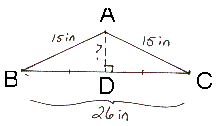​

Detailed Solution for Test: Problems on Pythagoras Theorem - Question 3

Height of the triangle is the altitude. SInce the triangle is an isosceles triangle its median is equal to its altitude . So this means that the altitude is perpendicular and bisects the opposite side .
So BD=DC=26/2=13
So in right angled Triangle,
Using Pythagoras theorem,
H= P+ B2
225 = P+ 169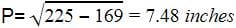Test: Problems on Pythagoras Theorem - Question 4

If the sum of the squares of a and b is the same as the square of c and if m < n, then for what value of k can the Pythagorean triples be generated with the following equations?
a = n2 + k , b = 2mn : c = m2 + n2

Test: Problems on Pythagoras Theorem - Question 5

Find the total perimeter of the given figure.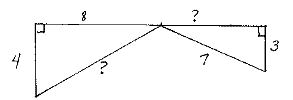Test: Problems on Pythagoras Theorem - Question 6

What is the diagonal length of a TV screen whose dimensions are 80 cm x 60 cm?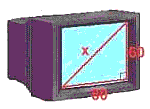Test: Problems on Pythagoras Theorem - Question 7

If the sum of the length of the legs of a right triangle is 49 cm and the hypotenuse is 41 cm, find its shortest side.​

Detailed Solution for Test: Problems on Pythagoras Theorem - Question 7

Sum of the length of the legs of a right angle triangle is 49
So let one leg be x, other will be 49-x
Applying Pythagoras theorem,
H2=P2+B2
41*41=x2+(49-x)2
1681=x+ 2401 + x- 98x
2x2- 98x + 720 = 0
Dividing the equation by 2
x- 49x + 360 = 0
x- 9x - 40x + 360 = 0
x (x - 9) - 40 (x - 9) = 0
(x - 9)(x - 40) = 0
So x is either 9 or 40,
So the smaller side is 9 cm

Test: Problems on Pythagoras Theorem - Question 8

How far up a wall will an 11m ladder reach, if the foot of the ladder is 4m away from the wall?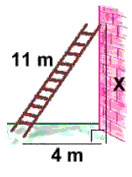Detailed Solution for Test: Problems on Pythagoras Theorem - Question 8

The height of wall will be the perpendicular for the right angle triangle. So applying pythagoras theorem
H2=P2+B2
11*11=P2+16
P=121-16=10.2 cm

Test: Problems on Pythagoras Theorem - Question 9

A wood frame for pouring concrete has an interior perimeter of 14 metres. Its length is one metre greater than its width. The frame is to be braced with twelve-gauge steel cross-wires. Assuming an extra half-metre of wire is used at either end of a cross-wire for anchoring, what length of wire should be cut for each brace?​

Detailed Solution for Test: Problems on Pythagoras Theorem - Question 9

Let width is x
Then length is x+1
Perimeter =x +x + x + 1 + x + 1 = 14
4 x= 12
x = 3
length = 4
Using Pythagoras theorem,
H2 = P+ B2
=9 + 16 = 25
H = 5cm
An extra half meter on either side will give the length of the wire as 5 + 1 = 6cm

Test: Problems on Pythagoras Theorem - Question 10

Anuj swims across a pool diagonally every day. The pool is 4 m wide, and it is 16 m diagonally across. What is the length of the pool?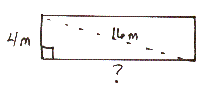## Online MCQ Tests for Class 10

615 tests
Information about Test: Problems on Pythagoras Theorem Page
In this test you can find the Exam questions for Test: Problems on Pythagoras Theorem solved & explained in the simplest way possible. Besides giving Questions and answers for Test: Problems on Pythagoras Theorem, EduRev gives you an ample number of Online tests for practice

615 tests